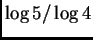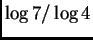MAT 331, Fall 2002
Project 3: Fractals
Due Wednesay, December 11

In this project, you are asked to define a fractal of your choice whose box-counting dimension is. Describe clearly how you construct such a set and prove that its dimension is the requested number. Also, plot the first 6 approximating curves to the fractal, using either a TurtleCmd procedure or IFS (see http://www.math.sunysb.edu/~mat331/Worksheets/IFS.mws).

As always, pay attention to clarity of exposition; and describe what you do at each step from a mathematical viewpoint, not as a commentary on how to use Maple. In your proof you can use the results we proved in class.

Bonus: Do the same thing for a fractal of box dimension.

MAT 331 2002-11-13